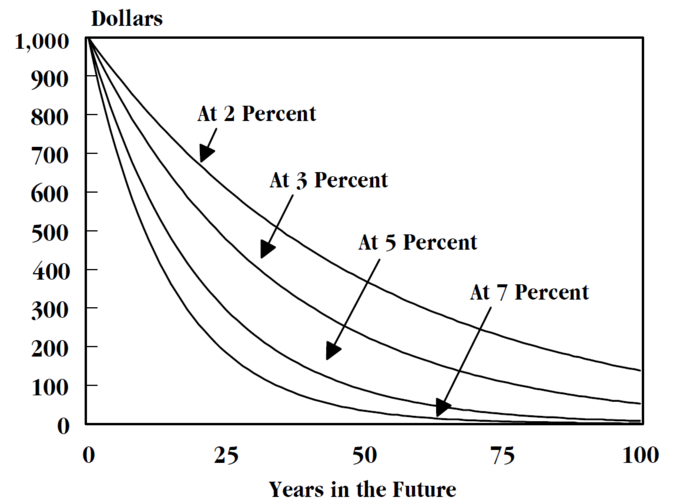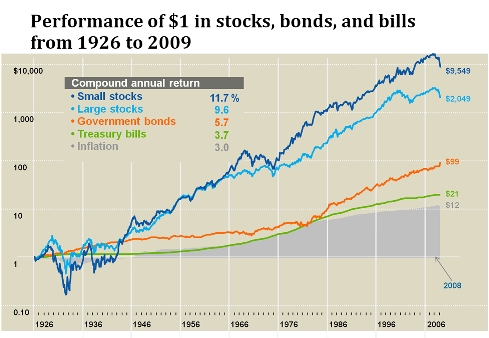## Risk Adjusting the Discount Rate

Discount rates are adjusted on an investment to investment basis, as different investments encounter different degrees of risk that must be considered when determining equitable returns.

### Learning Objectives

Realize the reasoning behind adjusting discount rates for risk, and the way this impacts the cost of capital

### Key Takeaways

#### Key Points

• In central banking, the established discount rate will act as the benchmark for future interest rates issued. This cost will be adjusted on an investment to investment basis depending upon the degree of risk.
• Discount rates are utilized in net present value calculations (NPV) in order to determine the rate of risk and return a new venture or new project should provide.
• Calculating NPV is an extremely useful tool. An NPV analysis will take future expectations of cost and revenue and ground them in present day dollars (to take into account the time value of money). This shows if/when a project will be profitable.
• When adjusting the NPV calculation for additional risks, and thus risk-adjusting the discount rate, a variety of factors can be taken into consideration. A rNPV (risk-adjusting NPV) will assign a probability to each incoming cash flow to adjust expectations of risk in the calculation.

#### Key Terms

• discounted cash flow: An estimated future cash flow discounted by the probability of receiving that cash flow.

### What is the Discount Rate?

The discount rate has a few definitions, depending on the context. For the sake of this discussion, the discount rate is the percentage used in an net present value calculation to understand the overall cost of capital (or, from the perspective of some investors, the required return) on a given project. What this means is that there is a rate, calculated by the assumption of risk, which is used to normalize the interest rate on borrowed capital (for the borrower) and/or invested capital (for the lender.

In U.S. central banking, the interest rate that banks pay the federal reserve when using securities as collateral is also referred to as the discount rate, and in this case it will act as the benchmark for future interest rates issued.

The primary purpose of a discount rate, or an interest rate in general, is fairly simple. Capital today is worth more than capital tomorrow, due to the time value of money (i.e. the opportunity cost of foregone investments). As a result of this concept, the idea of interest rates is justified. All this means is that a borrower of capital will have to take into account the cost of that capital over a given time period, which will be calculated as an additional percentage of the overall principal borrowed being added to the principal itself on a period to period basis.

In this context, the interest rate is subjected to risk. A riskier investment will require a higher return (due to the basic premise of risk and return). After all, why invest in a riskier investment when safer investments exist unless that riskier investment offers a higher percentage of return? It is at this point that the logic behind adjusting discount rates becomes practical. All discount rates must take into account the overall risk being assumed in the investment, and adjust the rate of expected return to meet the implications of the overall risk over time. For banks, for example, the overall interest rate they would offer a risky entrepreneurial project isn’t the same as the rate they would offer an established big business.

A net present value calculation is a common and useful tool that takes the overall future projected costs and returns of a new venture or business project and grounds it in today’s dollars. What this means is it calculates out the time value of money during the projected time period of the financing of the project in order to see if the returns over a given time period will exceed the costs in beginning the project. As an entrepreneur, this will tell you if/when you will reach profitability (using a variety of assumptions). It is calculated as follows:

$\mathrm {\text{NPV}} (\text{i},\text{N})=\sum _{\text{t}=0}^{\text{N}}{\frac {\text{R}_{\text{t}}}{(1+\text{i})^{\text{t}}}}}$

Adjusting this for the risk-adjusted discount rate is a simple modification, where each future cash flow is multiplied by the estimated likelihood of its occurrence. In this situation, a higher degree of uncertainty (and thus risk) is built into each expected cash flow (called a discounted cash flow, or DCF). Through discounting each cash flow by the estimated probability of receiving that return, the overall riskiness of the NPV calculation is increased. With this increase in risk, the discount rate can now be risk-adjusted accordingly.

It is important to note at this point that every calculation is different, and some start-ups will see low discount rates because investors believe strongly in what the start up is trying to accomplish. However, the discount rates typically applied to different types of companies show significant differences:

• Early start-ups: 40–60%
• More established start-ups: 30–50%
• Mature companies: 10–25%

All and all, investors must carefully consider the risk in a given investment, and adjust the discount rates accordingly. This ensures a proper assessment of the risk and return ratio for various differentiated investment projects.Discounting Curves: This chart illustrates the devaluation of capital over time as a result of various discount rates.

## Risk Adjusting for the Time Horizon

A longer time horizon usually requires a higher return, due to increased price volatility and uncertainty relating to possible outcomes.

### Learning Objectives

Explain how an investement’s duration can influence its inherent risk

### Key Takeaways

#### Key Points

• In terms of debt investments, default risk increases as the time horizon lengthens.
• Since stock investments have more time to overcome potential downturns in value, having a longer time horizon can justify more aggressive investing.
• When there are multiple possible outcomes, management of a firm may choose to undertake real options analysis in order to factor in the various possibilities.

#### Key Terms

• time horizon: The period of time the asset is expected to be held or a project is expected to last.
• salvage value: The estimated value of an asset at the end of its useful life.

### Risk Adjusting for the Time Horizon

When evaluating the riskiness of an investment, not only will investors or companies need to evaluate their preferences and risk tolerance, but it is also necessary to take into account the time horizon of the investment. A longer time horizon will generally require a higher return, due to an increased risk in price volatility and increased uncertainty relating to possible outcomes. In terms of long term debt investments, such as long term corporate or government bonds, a longer time horizon gives rise to uncertainties in the potential operations of the debtor entity as well as unforeseen movements in the market as a whole. In other words, default risk increases as the time horizon lengthens. However, when considering stock investments, having a longer time horizon can be considered safer in some respects. Since stock investments have more time to overcome potential downturns in value, having a longer time horizon can justify more aggressive investing. For example, an individual investor with a time horizon of less than five years should not be invested in stocks. However, those with time horizons over five years should consider stocks because of their growth potential. Put simply, as time horizon lengthens a higher percentages of stocks should be added to a portfolio.Performance of Stocks, Bonds and Bills: This graph gives an illustration of investing a dollar in different asset classes in the market from 1926 to the end of 2007.

For an individual, diversifying investments in different time horizons is also important. By staying in the market through different market cycles, individuals can reduce the risk of receiving a lower return than expected–especially with investments that fluctuate significantly over the short term.

### Real Options Consideration

When there are multiple possible outcomes, management of a firm may choose to undertake real options analysis in order to factor in the various possibilities. A real option itself is the right, but not the obligation, to undertake certain business initiatives, such as deferring, abandoning, expanding, staging, or contracting a capital investment project. When uncertainty exists as to when and how business or other conditions will eventuate, flexibility as to the timing of the relevant project is valuable and constitutes optionality. Some examples include Inflation or deferment options, where management has flexibility as to when to start a project. For example, in natural resource exploration a firm can delay mining a deposit until market conditions are favorable. Also, an option to abandon, where management may have the option to cease a project during its life, and, possibly, to realize its salvage value. Here, when the present value of the remaining cash flows falls below this salvage value, the asset may be sold. Finally, sequencing options, where management can observe outcomes from a part of a project and resolve some of the uncertainty relating to the venture overall. Once resolved, management has the option to proceed or not with the development of the other projects.